# GCSE Non-Calculator Questions 1 (2013/Higher Tier)

This worksheet contains a sample of practice questions based on the 2013 GCSE Higher Level syllabus. Calculators may not be used.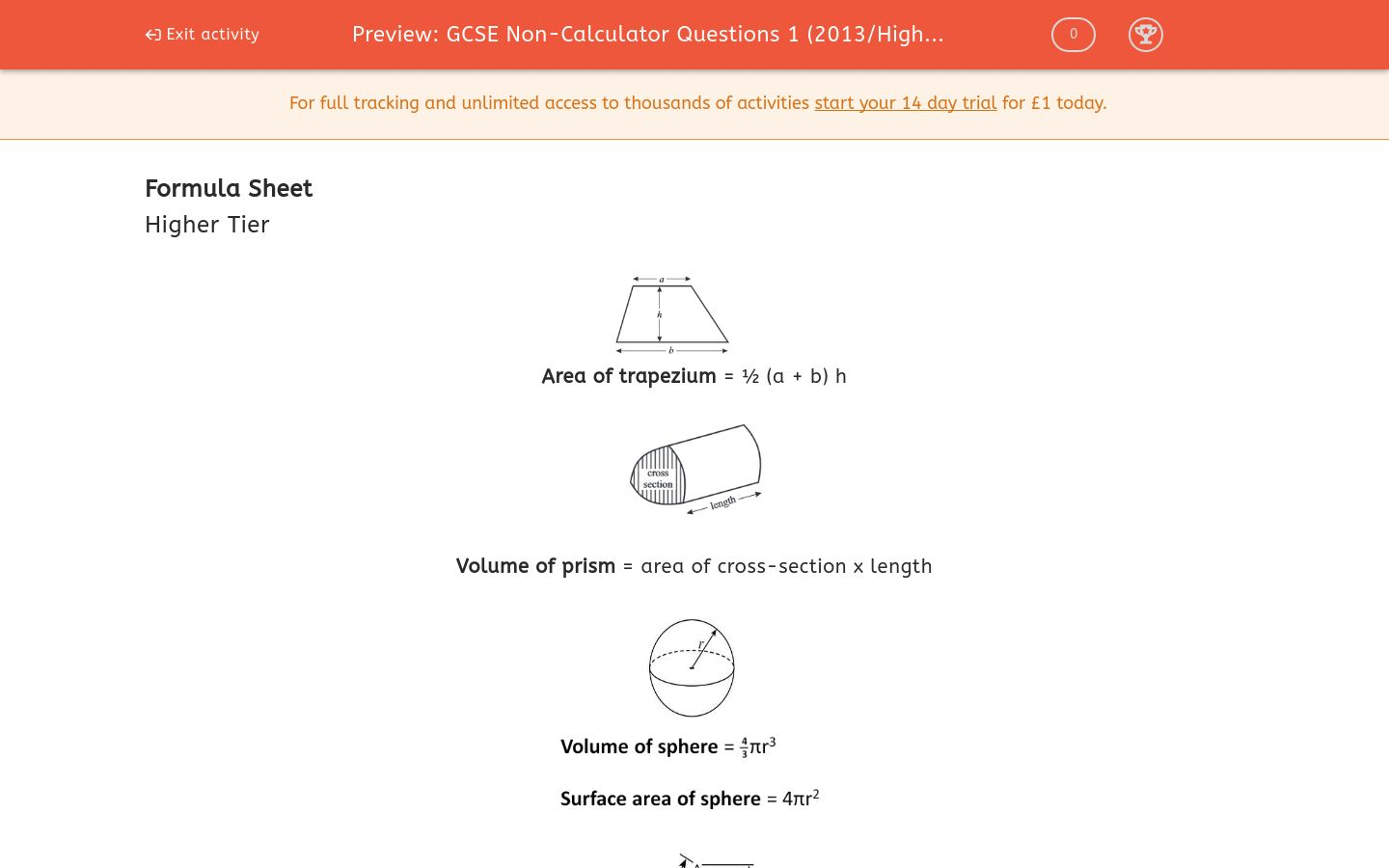Key stage:  KS 4

Curriculum topic:  GCSE Practice Papers

Curriculum subtopic:  Selection of Topics for Non-Calculator Practice

Difficulty level:### QUESTION 1 of 10

Formula Sheet

Higher TierArea of trapezium = ½ (a + b) hVolume of prism = area of cross-section x length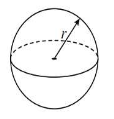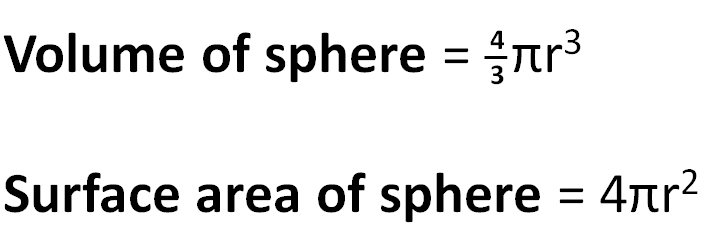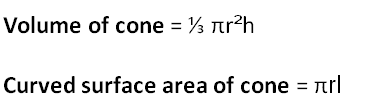In any triangle ABC:

Area of triangle = ½ab sinC

Sine rule

 a = b = c sin A sin B sin C

Cosine rule

a2 = b2 + c2 - 2bc cos A

The solutions of ax2 + bx + c = 0, where a ≠ 0, are given byWork out:

 3 - 1 8 5

Work out:

 3 x 8 12 15

(Give your answer as a fraction in its lowest terms in the form a/b)

Work out the reciprocal of

0.125

Expand

5(a - 3)

Factorise fully

5a2 - 15a

Anna wishes to lay carpet tiles on her floor, a plan of which is shown below.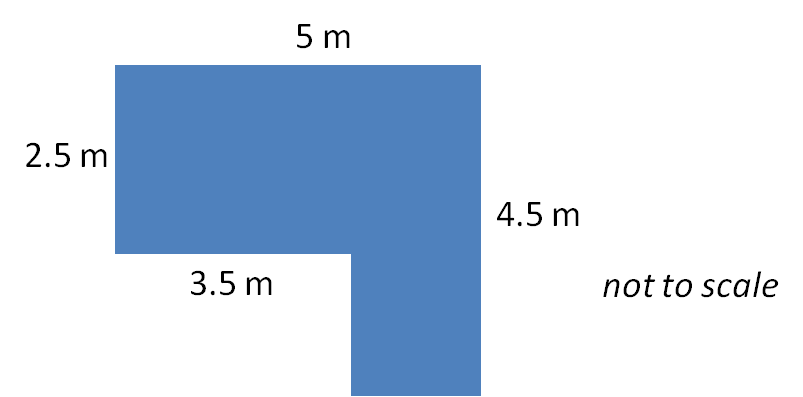Each carpet tile is a square of side length 25 cm and they are sold in packs of 12.

Each pack costs £12.

How much will Anna have to spend on carpet tiles to tile her L-shaped room?

(Just write the number of £s)

PQ is parallel to RS.

Triangle ABC is isosceles with AB = BC.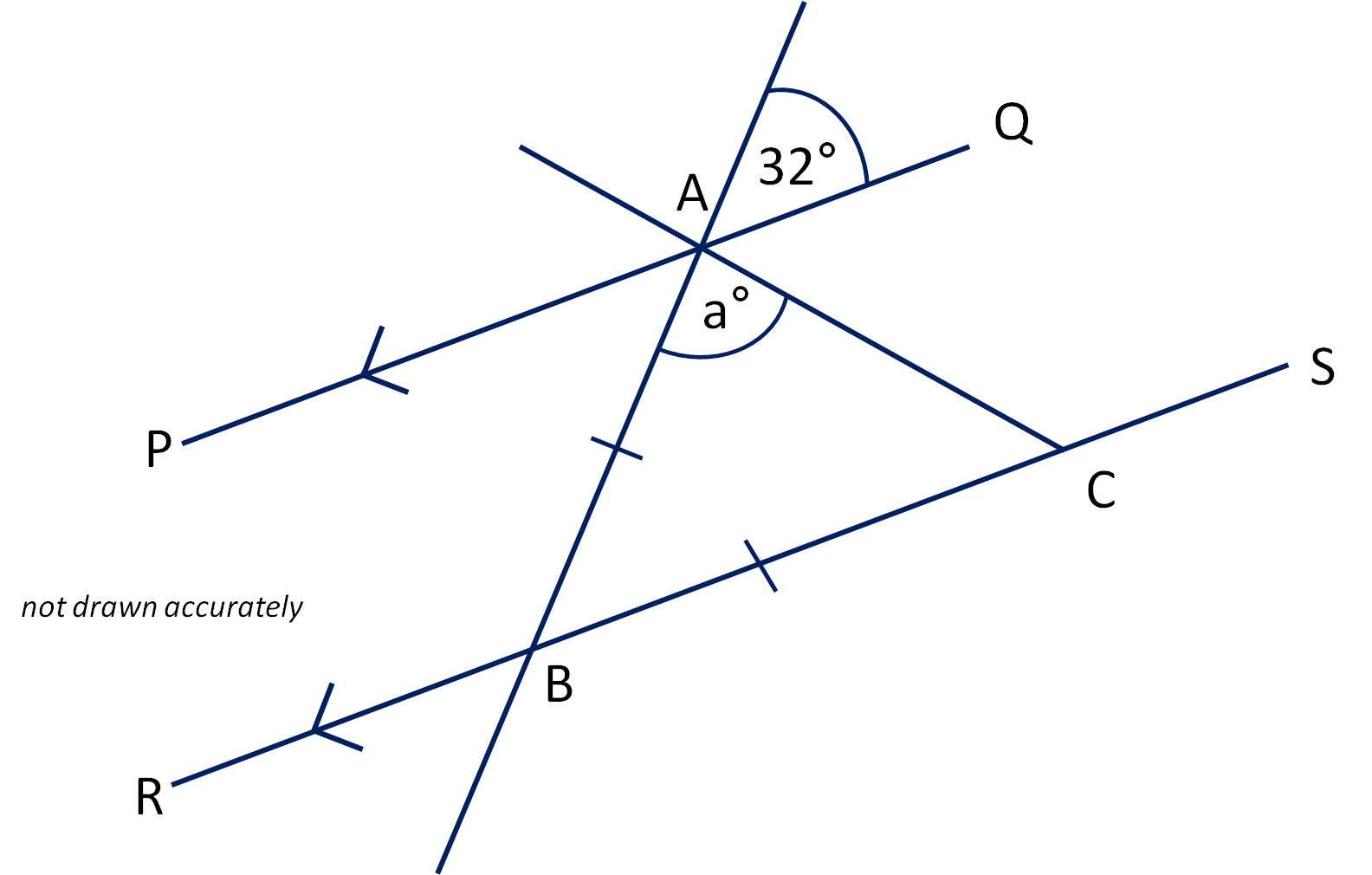Calculate the value of a.

(just write the number)

PQ is parallel to RS.

Triangle ABC is isosceles with AB = BC.Complete the sentence:

∠PAB and ∠ABC are...

opposite

interior

alternate

corresponding

Solve for x:

15x - 4 = 10x + 26

(just write the value of x as a number)

Expand and simplify:

6(x - 2) - 4(x - 5)

2x - 32

10x - 32

2x + 8

2x - 7

The bearing of B from A is 061°.

What is the bearing of A from B?

(just write the number of degrees)

Andy drives along a motorway in France at an average speed of 96 km/h for 2 hours 15 minutes.

How many miles does Andy drive?

Write down the value of

641/3

Write down the value of

64

(write as a decimal)

The interior angle of a regular polygon is 160°.

Calculate how many sides the polygon has.

Simplify

√28 + √63

5√14

7√14

5√7

13 +4√7

√91

Last year, a bicycle cost £160.

This year, the price of the same model of bicycle has increased by 15%.

Calculate the price of the bicycle this year in £s.

(Just write the number)

Work out the value of the following expression, giving your answer in standard form:

5 x 103 + 2 x 105

10 × 1035

2.05 × 105

2.05 × 103

1 × 105

10 × 1015

State the coordinates of the midpoint of the line segment whose endpoints are at

(6, 3) and (-2, -6)

(use decimals if you have to)

The first five terms of a number sequence are:

7      13      19      25      31 ...

Write down an expression, in terms of n, for the nth term of the sequence.

Two similar solid shapes, A and B, are shown below.Solid B has a volume of 120 cm3.

Calculate the volume of solid A in cm3.

(Just write the number)

There are 7 red balls and 5 yellow balls in a bag.

I take one ball from the bag and keep it.  I then take another ball from the bag.

What is the probability of choosing a red ball and a yellow one?By completing the square, write the following in the form (x + a)2 + b, where a and b are integers.

x2 + 10x + 31

(x + 3)² + 25

(x + 10)² + 6

(x + 20)² + 31

(x + 10)² + 25

(x + 5)² + 6

Factorise

x2 - 16

(x - 4) (x - 4)

(x - 4) (x + 4)

(x - 8) (x - 2)

(x - 256) (x + 256)

(x - 4)²

Estimate the value of the following to 1 significant figure:

 5.962 - √15.9) 1.983

• Question 1

Work out:

 3 - 1 8 5

7/40
EDDIE SAYS
Change to 40ths to get:
 15 - 8 40 40
• Question 2

Work out:

 3 x 8 12 15

(Give your answer as a fraction in its lowest terms in the form a/b)

2/15
EDDIE SAYS
Reduce within the fraction to get:
 1 × 2 3 5

Then multiply the tops and the bottoms separately.
• Question 3

Work out the reciprocal of

0.125

8
EDDIE SAYS
0.125 = 1/8
The reciprocal of 1/8 is 8
• Question 4

Expand

5(a - 3)

5a-15
EDDIE SAYS
Multiply a by 5 and then multiply -3 by 5
• Question 5

Factorise fully

5a2 - 15a

5a(a-3)
EDDIE SAYS
5a is the common factor.
Divide both terms by 5a to work out what goes inside the brackets.
• Question 6

Anna wishes to lay carpet tiles on her floor, a plan of which is shown below.Each carpet tile is a square of side length 25 cm and they are sold in packs of 12.

Each pack costs £12.

How much will Anna have to spend on carpet tiles to tile her L-shaped room?

(Just write the number of £s)

252
EDDIE SAYS
25 cm squares will tessellate the room, without the need to cut any of the square tiles.
16 tiles will cover an area of 1 m²
Area of room = 5 × 4.5 - 3.5 × 2 = 15.5 m²
So she will need 15.5 × 16 = 248 tiles.
20 packs will give her 240 tiles, so she needs 21 packs, costing 21 × 12 = £252.
• Question 7

PQ is parallel to RS.

Triangle ABC is isosceles with AB = BC.Calculate the value of a.

(just write the number)

74
EDDIE SAYS
∠ABC corresponds to the angle shown as 32° and so ∠ABC = 32°
a = ½(180 - 32) = 74
• Question 8

PQ is parallel to RS.

Triangle ABC is isosceles with AB = BC.Complete the sentence:

∠PAB and ∠ABC are...

alternate
EDDIE SAYS
They form a Z shape on parallel lines.
• Question 9

Solve for x:

15x - 4 = 10x + 26

(just write the value of x as a number)

6
EDDIE SAYS
15x - 4 = 10x + 26
Subtract 10x from both sides to get:
5x - 4 = 26
Add 4 to both sides to get:
5x = 30
Divide both sides by 5 to get:
x = 6
• Question 10

Expand and simplify:

6(x - 2) - 4(x - 5)

2x + 8
EDDIE SAYS
6x - 12 - 4x + 20
2x + 8
• Question 11

The bearing of B from A is 061°.

What is the bearing of A from B?

(just write the number of degrees)

241
EDDIE SAYS• Question 12

Andy drives along a motorway in France at an average speed of 96 km/h for 2 hours 15 minutes.

How many miles does Andy drive?

135
EDDIE SAYS
96 km = 96 ÷ 8 × 5 = 60 miles
Time = 2 hours 15 minutes = 2¼ hours = 2.25 hours
Distance = Speed × Time = 60 × 2.25 = 135 miles
• Question 13

Write down the value of

641/3

4
EDDIE SAYS
4³ = 64
• Question 14

Write down the value of

64

(write as a decimal)

0.125
EDDIE SAYS
64½ = √64 = 8
The negative index tells you to find the reciprocal of 8, which equals 1 ÷ 8 = 0.125
• Question 15

The interior angle of a regular polygon is 160°.

Calculate how many sides the polygon has.

18
EDDIE SAYS
The exterior angle = 180° - 160° = 20°
Number of sides = 360 ÷ 20 = 18
• Question 16

Simplify

√28 + √63

5√7
EDDIE SAYS
= √(4 × 7) + √(9 × 7)
= √4 × √7 + √9 × √7
= 2√7 + 3√7
= 5√7
• Question 17

Last year, a bicycle cost £160.

This year, the price of the same model of bicycle has increased by 15%.

Calculate the price of the bicycle this year in £s.

(Just write the number)

184
EDDIE SAYS
160 × 1.15 = 184
• Question 18

Work out the value of the following expression, giving your answer in standard form:

5 x 103 + 2 x 105

2.05 × 105
EDDIE SAYS
5 × 103 = 5000
2 × 105 = 200000
Add to get 205000 = 2.05 × 105
• Question 19

State the coordinates of the midpoint of the line segment whose endpoints are at

(6, 3) and (-2, -6)

(use decimals if you have to)

(2, -1.5)
(2,-1.5)
EDDIE SAYS
The x-coordinate is ½(6 + -2)
The y-coordinate is ½(3 + -6)
• Question 20

The first five terms of a number sequence are:

7      13      19      25      31 ...

Write down an expression, in terms of n, for the nth term of the sequence.

6n+1
EDDIE SAYS
The gap between each term is 6, so the expression will have a 6n in it.
6n would give the sequence 6, 12, 18, 24, 30 etc.
The given sequence is one more than this.
• Question 21

Two similar solid shapes, A and B, are shown below.Solid B has a volume of 120 cm3.

Calculate the volume of solid A in cm3.

(Just write the number)

15
EDDIE SAYS
Ratio of lengths = 3:6 = 1:2
Ratio of volumes = 1³:2³ = 1:8
120 cm³ ÷ 8 = 15 cm³
• Question 22

There are 7 red balls and 5 yellow balls in a bag.

I take one ball from the bag and keep it.  I then take another ball from the bag.

What is the probability of choosing a red ball and a yellow one?35/66
EDDIE SAYS
We can choose red then yellow, or yellow then red.
Probability = 7/12 × 5/11 + 5/12 × 7/11 = 70/132, which reduces to 35/66.
• Question 23

By completing the square, write the following in the form (x + a)2 + b, where a and b are integers.

x2 + 10x + 31

(x + 5)² + 6
EDDIE SAYS
(x + 5)² = (x + 5)(x + 5) = x² + 10x + 25
We must add 6 to get to 31.
• Question 24

Factorise

x2 - 16

(x - 4) (x + 4)
EDDIE SAYS
This is the difference of two squares.
√16 = 4
(x - 4)(x + 4) is the factorisation.
• Question 25

Estimate the value of the following to 1 significant figure:

 5.962 - √15.9) 1.983

4
EDDIE SAYS
5.96² ≅ 6² = 36
√15.9 ≅ √16 = 4
1.98³ ≅ 2³ = 8
So we have (36 - 4) ÷ 8 = 32 ÷ 8 = 4
---- OR ----

Sign up for a £1 trial so you can track and measure your child's progress on this activity.

### What is EdPlace?

We're your National Curriculum aligned online education content provider helping each child succeed in English, maths and science from year 1 to GCSE. With an EdPlace account you’ll be able to track and measure progress, helping each child achieve their best. We build confidence and attainment by personalising each child’s learning at a level that suits them.